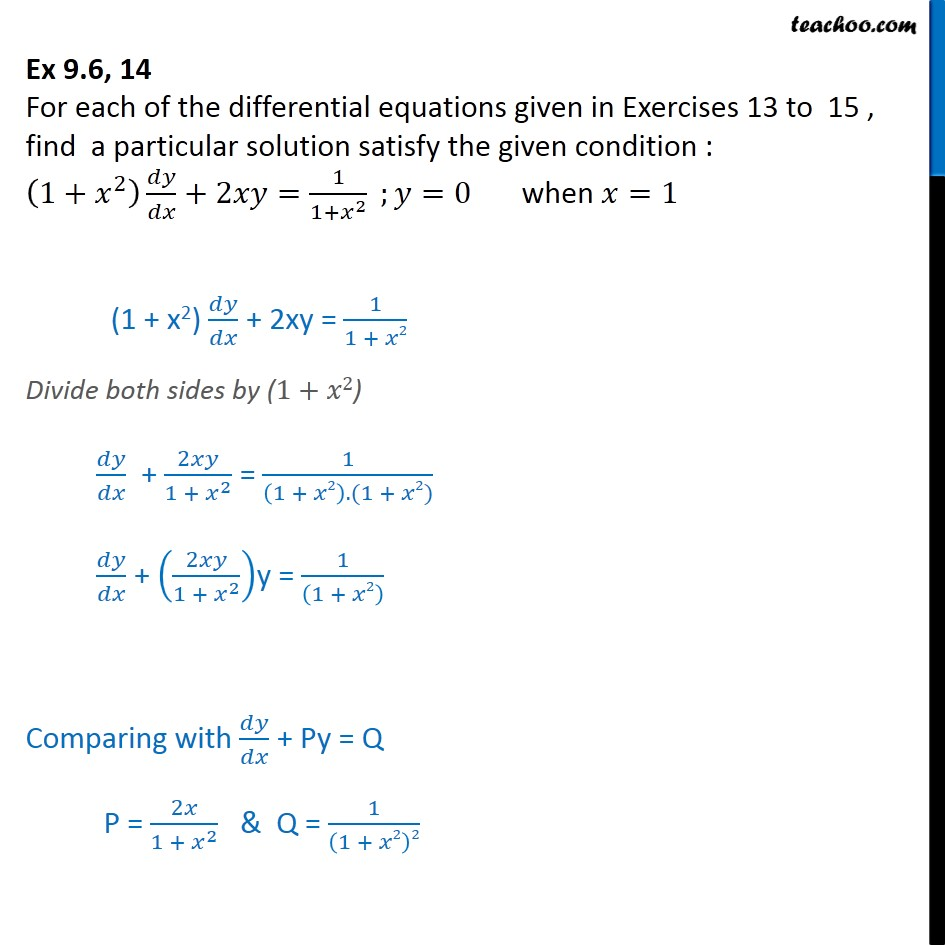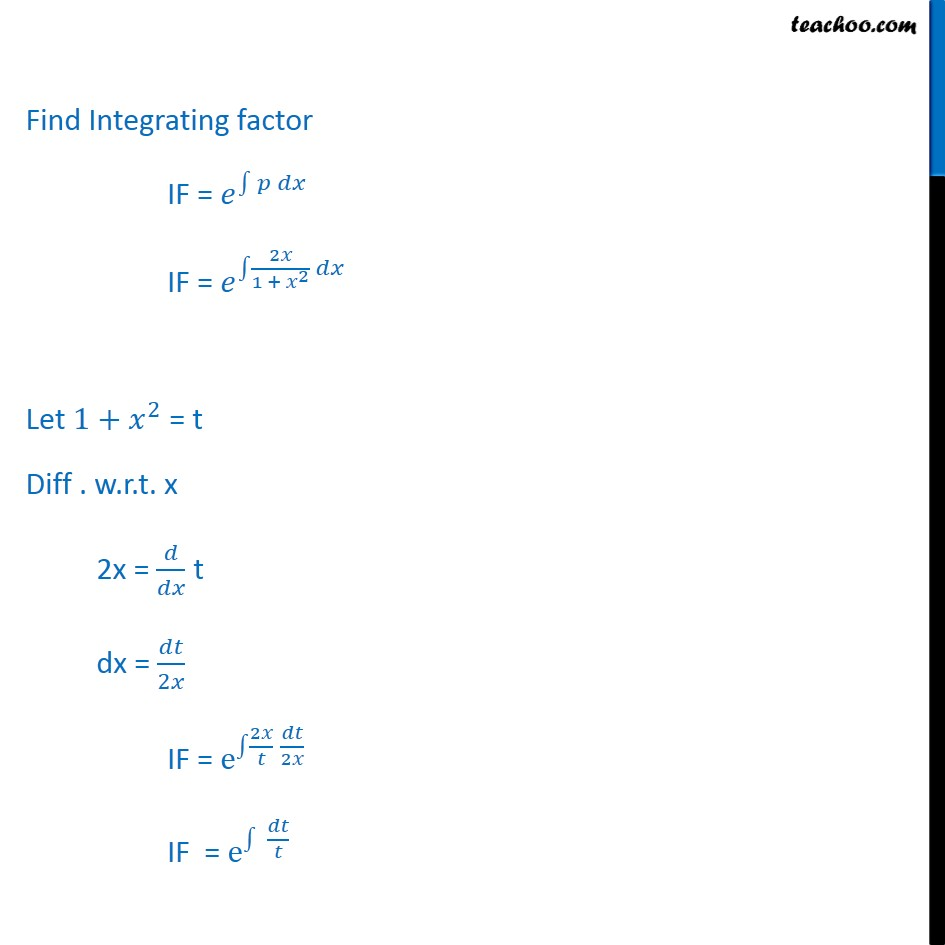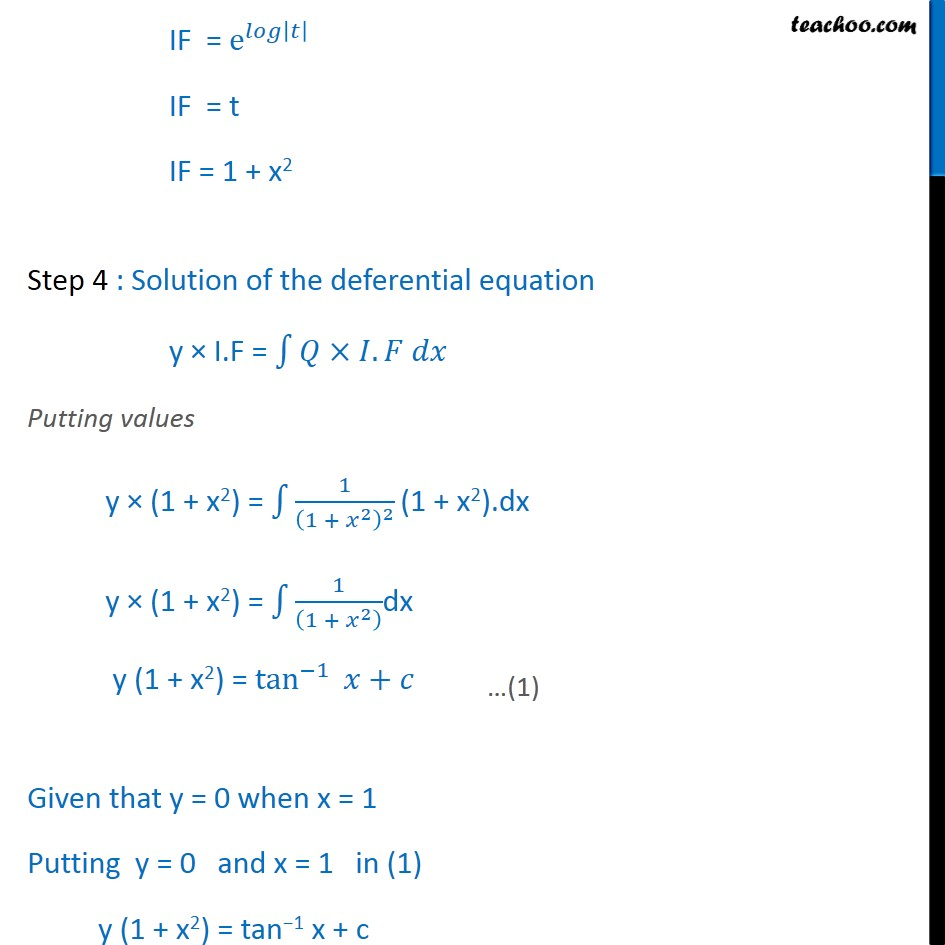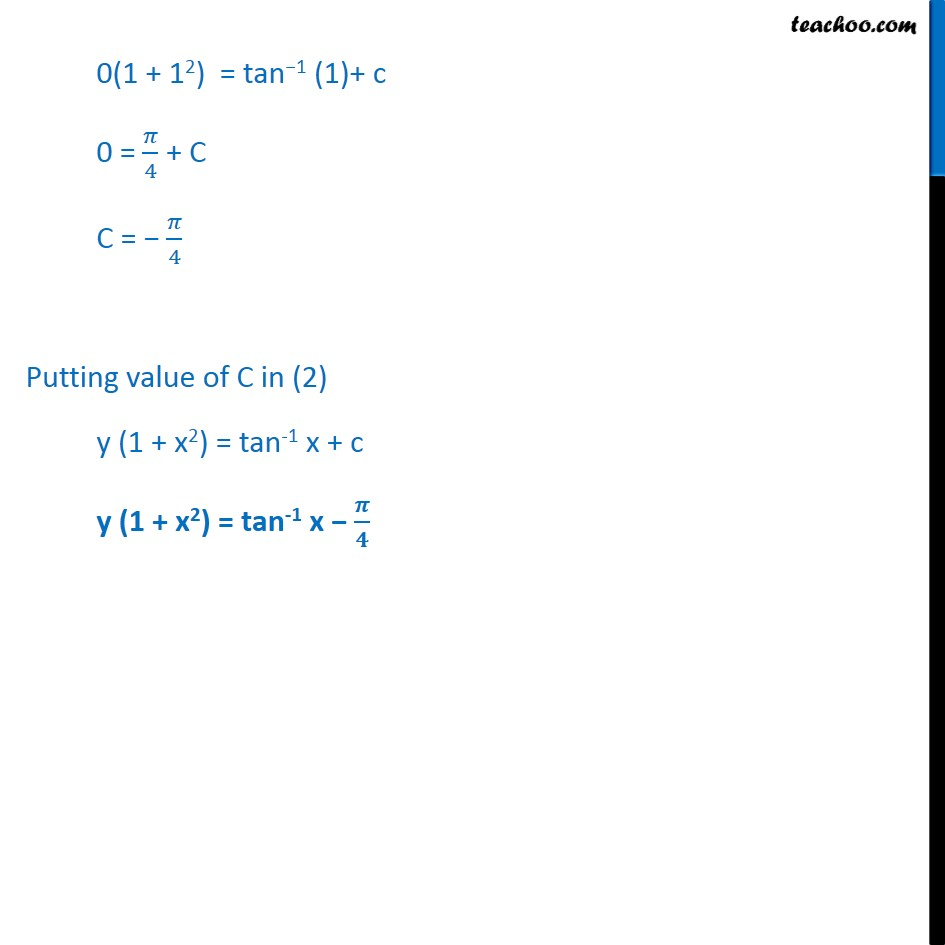Ex 9.5

Chapter 9 Class 12 Differential Equations
Serial order wiseLearn in your speed, with individual attention - Teachoo Maths 1-on-1 Class

### Transcript

Ex 9.5, 14 For each of the differential equations given in Exercises 13 to 15 , find a particular solution satisfy the given condition : 1+ 2 +2 = 1 1+ 2 ; =0 when =1 (1 + x2) + 2xy = 1 1 + 2 Divide both sides by (1+ 2) + 2 1 + 2 = 1 1 + 2 .(1 + 2) + 2 1 + 2 y = 1 1 + 2 Comparing with + Py = Q P = 2 1 + 2 & Q = 1 1 + 2 2 Find Integrating factor IF = IF = 2 1 + 2 Let 1+ 2 = t Diff . w.r.t. x 2x = t dx = 2 IF = e 2 2 IF = e IF = e IF = t IF = 1 + x2 Step 4 : Solution of the deferential equation y I.F = . Putting values y (1 + x2) = 1 1 + 2 2 (1 + x2).dx y (1 + x2) = 1 1 + 2 dx y (1 + x2) = tan 1 + Given that y = 0 when x = 1 Putting y = 0 and x = 1 in (1) y (1 + x2) = tan 1 x + c 0(1 + 12) = tan 1 (1)+ c 0 = 4 + C C = 4 Putting value of C in (2) y (1 + x2) = tan-1 x + c y (1 + x2) = tan-1 x